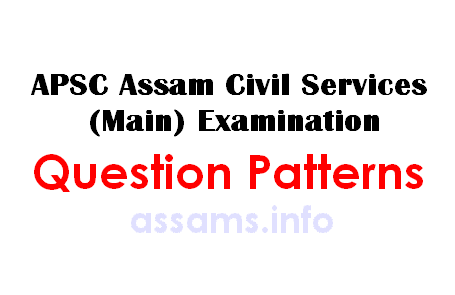# APSC Combined Competitive (Main) Examination Question Patterns

Assam Public Service Commission (APSC) has released the question pattern for Combined Competitive (Main) Examination, 2014. The mark wise breakup of question paper is different for different subjects. This would help the candidates, specially the first timers. The original notification containing the question pattern can be downloaded from official APSC website (find the download link at the end of this page). For the benefit of candidates, we are publishing the question pattern here on assams.info. As the released question pattern is all about marks, number of questions and word limit, we tried to create a simplified version. And the result is following:## APSC CC (Main) Exam Question Patterns:

Subjects: MIL (Assamese, Bengali, English, Hindi, Arabic, Persian, Sanskrit and Bodo), Anthropology, Commerce, Economics, Education, History, Law, Philosophy, Political Science, Psychology, Sociology, Statistics, Agriculture, Animal Husbandry & Veterinary Science, Botany, Chemistry, Fisheries, Geology, Geography, Mathematics, Medical Science, Physics and Zoology

a) 20 markers: Total 12 questions, out of which 8 to be answered. Word limit is 250 words. Total marks: 8×20 = 160
b) 8 markers: Total 8 questions, out of which 5 to be answered. Word limit is 150 words. Total marks: 5×8= 40

Grand total = 200 marks (each paper)

• In case of the MIL Subjects, one essay type question may be included. Such questions would carry 20 marks and are to be answered within 250 words.
• The 20 marker questions may have sub-questions of maximum 3 parts. Marks for each sub question will be indicated after the question (Example: 10+5+5=20).

Subjects: Chemical Engineering, Civil Engineering, Computer Science, Electrical Engineering, Electronics and Mechanical Engineering.

a) 20 markers: Total 15 questions, out of which 10 to be answered. No word limit. Total marks: 10×20 = 200

Grand total = 200 marks (each paper)

• Each question will have sub questions containing up to 3 parts. Marks for each sub question will be indicated after the question (Example: 10+5+5=20).

Subject: General English

a) Precis writing: 40 marks. The word limit should be one third of the given passage
b) Essays writing (2 Nos.): 50 marks each. Word limit is 400-500 words.
c) Comprehension: 50 marks.
d) One paragraph on current topic (new addition): 10 marks. Word limit is 150 words.
e) Amplification: 20 marks. Word limit is 150-200 words.
f) Grammar: 50 marks.
g) Reporting writing & letter writing: 30 marks. Word limit is 200-250 words

Grand total = 300 marks.

Subject: General Studies

a) 16 markers: Total 15 questions, out of which 10 are to be answered. Word limit is 200 words each question. Each question will have sub question of maximum 2. Total marks: 16×10 = 160.
b) 8 markers: Total 8 questions, out of which 5 are to be answered. Word limit is 100 words each question. Each question will have sub question of maximum 2. Total marks: 8×5 = 40
c) 5 markers: Total 12 questions, out of which 8 are to be answered. Word limit is 50 words. Total marks: 5×8 = 40
d) 2 markers: Total 35 questions, out of which 30 are to be answered. Total marks: 2×30 = 60

Grand total = 300 marks

Note: The APSC notification states it as a probable question pattern. So, don’t be surprised if there is slight deviation from the stated pattern. All the best.